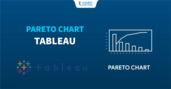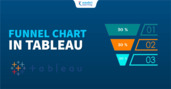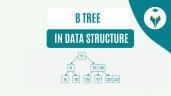Dual Axis Chart in Tableau

# Dual Axis Chart in Tableau

clickHere
Updated on May 11, 2022 17:59 IST

In this article we will discuss one of the type of chart in tableau i.e. Dual Axis Chart in Tableau with an example.

## Introduction

In this article, we will discuss the Dual Axis chart in the tableau.

Tableau is a data visualization tool that allows drawing different charts and graphs using Show me with just simple drag and drop.

Tableau Show Me has 24 different charts.

Each chart in the tableau corresponds to a minimum number of dimensions, measures, bins, etc.

## Table of Content

There are 3 ways to compare multiple measures in a single view:

• Individual axes for each measure
• Blend two-measure two share an axes
• Add dual axes where two independent axes are layered in the same pane

## What is a Dual Axis Chart?

Dual-axis charts are a combination of two charts. It is used to visualize two or more different measures in two different chart types i.e. it depicts the relationship between two variables with different amplitude and scale.

To create a dual-axis chart we must have one date column and two measures.

Let’s understand how to create a Dual Axis Chart in Tableau with an example

Create a dual-axes chart of discount and profit for categories.Types of Charts in Tableau
In this article, we will discuss different types of charts in Tableau: Bar, Line, Gantt, Histogram, Tree, Box and Whisker Plot.Pareto Chart in Tableau
In this article we will discuss one of the type of chart in tableau i.e. Pareto Chart with an example.Bar and Line Chart in Tableau
In this article we will discuss how to create bar, stacked bar, side-by-side and line charts in Tableau with examples.

## Steps to create a Dual Axis Chart

• Connect a file (here, we will use Sample SuperStore data)
• Drag and Drop Category into the column shelf
• Drag and Drop Profit and Discount into the row shelf
• Here, we have two charts present on multiple axes
• Right-click on Sum (Profit)
• Select Dual-axis from the drop-down
• We get the desired dual-axis chart

Note: The above graph is a stacked bar graph. We can make it a bar and line (or whatever graph you want) graph in a few steps.

• In the Mark pane, you will get SUM(Discount) and SUM(Profit)
• Select the required chart from the drop-down
• In SUM(Discount) select Bar chart
• In SUM(Profit) select Line ChartFunnel Chart in Tableau
In this article we will discuss one of the type of chart in tableau i.e. Funnel Chart in Tableau with an example.Donut Chart in Tableau
In this article we will discuss one of the type of chart in tableau i.e. Donut Chart in Tableau with an example.Box and Whisker Chart in Tableau
In this article we will discuss one of the type of chart in tableau i.e. Box and Whisker Chart in Tableau with an example.

## Conclusion

In this article, we have discussed the Dual Axis Chart in Tableau with an example.

Through a series of articles, we will cover all the topics in-depth with examples.

clickHere

Vikram has a Postgraduate degree in Applied Mathematics, with a keen interest in Data Science and Machine Learning. He has experience of 2+ years in content creation in Mathematics, Statistics, Data Science, and Mac... Read Full Bio

## Trending Management CoursesVisual Analytics with Tableau
Coursera5.0Tableau Tutorial for Beginners
UDEMY4.5## Top Picks & New Arrivals